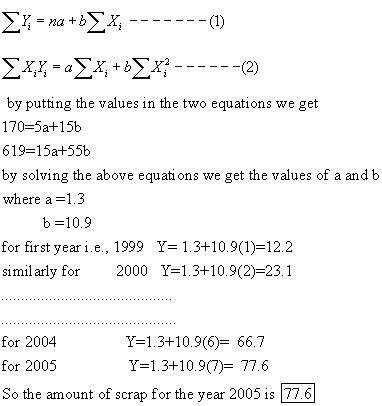# The following table lists the annual amounts of glass cullet produced by Kimble Glass Works, Inc. Year Code Scrap (tons) 1999 1 2.0 2000 2 4.0 2001 3 3.0 2002 4 5.0 2003 5 6.0 Determine the least squares trend equation. Estimate the amount of scrap for the year 2005.arenceabigns 2021-04-25 Answered

The following table lists the annual amounts of glass cullet produced by Kimble Glass Works, Inc.

$$\begin {array}{|c|c|} \hline \text{Year}&\text{Code}&\text{Scrap (tons)}\\ \hline 1999&1&2.0\\ \hline 2000&2&4.0\\ \hline 2001&3&3.0\\ \hline 2002&4&5.0\\ \hline 2003&5&6.0\\ \hline \end {array}$$

Determine the least squares trend equation. Estimate the amount of scrap for the year 2005.

• Questions are typically answered in as fast as 30 minutes

### Plainmath recommends

• Get a detailed answer even on the hardest topics.
• Ask an expert for a step-by-step guidance to learn to do it yourself.Nathaniel Kramer

First we have to draw a graph by then we can observethat this graph shows a straight line. So we have tofit a strainght line
Y= a+bX
where y is the scrap in tons and X is the years, take years as 1, 2, 3, 4, and 5 then
$$\begin{matrix}12&1&12&1\\24&2&48&4\\33&3&99&9\\45&4&180&16\\56&5&280&25\end{matrix}$$
total
$$\begin{matrix}170&15&619&55\end{matrix}$$
we know that the normal equations for straight line are